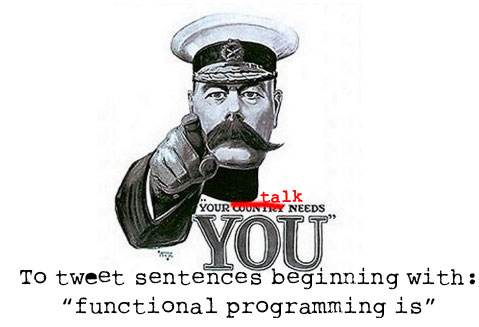_ _ _ ___ _ _ _ _ /_\ | |_ __ ___ __| |_ / _ \| |__ ___ ___| |___| |_ ___ _ _ ___| |_ / _ \| | ' \/ _ (_-< _| (_) | '_ (_-</ _ \ / -_) _/ -_)_| ' \/ -_) _| /_/ \_\_|_|_|_\___/__/\__|\___/|_.__/__/\___/_\___|\__\___(_)_||_\___|\__|
Functional JavaScript
(and some Underscore.JS too)

Thomas Parslow

tom@almostobsolete.net
@almostobsolete
http://almostobsolete.net

### Audience ParticipationFunctional programming is awesome.

Functional programming is a duck billed platypus

### Coming up!

• Why do functional programming in JavaScript?
• JavaScript is a functional language!
• Underscore.JS's functional helpers

### Why use a Functional style in JavaScript?

• Testable and Debugable
• Composable
• Less Code
• You can mix and match, it's fine to mix FP with OOP.

### JavaScript is Functional: Functions are First Class

• Functions in JavaScript are First Class Values
• Can be stored in variables, and passed into other functions

```function hello(talker) {
talker("Hello world");
}
function talk(text) {
output(text);
}
function shout(text) {
output(text.toUpperCase());
}
hello(talk);
hello(shout);
```

### JavaScript is Functional: Anonymous Functions

• Not every functions deserves a name
• Anonymous functions can be created on the spot and used right away
```function hello(talker) {
talker("Hello world");
}

hello(function (text) {
output('I am an anonymous function and I say: ' + text);
});
```

### JavaScript is Functional: Functions are Closures

• A JavaScript function is also a closure
• If you define a function inside another function you can access variables defined in the outer function from the inner one.
```function counter() {
var count = 0;
return function () {
return count++
};
}

var a = counter(), b = counter();
output('a()=', a());
output('a()=', a());
output('b()=', b());
```

### Underscore.JS

• A very useful library of utilities
• None are very complicated, but it's nice to have a single version of each.
• This is what Functional Programming is really good for, abstracting out little pieces of functionality: Re-use in the small.

### Map

```var animals = ['llama','alpaca','lemming']
function makeSentence(a) {
return a.toUpperCase() + a.slice(1) + ' is a type of animal';
} ```
Imperative:
```var results = [];
for(var i = 0; i < animals.length; i++) {
var animal = animals[i];
results.push(makeSentence(animal));
} ```
Functional:
```var results = _.map(animals, makeSentence);
output('Map results: ',results); ```

### Filter

```var animals = ['llama','alpaca','lemming']
function startsWithL(a) {
return a === 'l';
} ```
Imperative:
```var results = [];
for(var i = 0; i < animals.length; i++) {
var animal = animals[i];
if (startsWithL(animal)) {
results.push(animal);
}
} ```
Functional:
```results = _.filter(animals, startsWithL);
output('Filter output:', results);```

### Reduce

```var animals = ['llama','alpaca','lemming']
return length+string.length;
} ```
Imperative:
```var result = 0;
for(var i = 0; i < animals.length; i++) {
var s = animals[i];
} ```
Functional:
```result = _.reduce(animals, addLength, 0);
output('Reduce output:', result);```

### Zip

```var animals = ['llama','alpaca','lemming'];
var names = ['larry','al','lem']; ```
Imperative:
```var results = [];
for(var i = 0; i < animals.length; i++) {
var animal = animals[i], name = names[i];
results.push([animal, name]);
}
```
Functional:
```results = _.zip(animals, names);
output('Zip output:', results);```

### Zip and Map together

```var animals = ['llama','alpaca','lemming'];
var names = ['larry','al','lem'];
function nameAnimal(pair) {
return pair + ' the ' + pair;
} ```
Imperative:
```var results = [];
for(var i = 0; i < animals.length; i++) {
var name = names[i], animal = animals[i];
results.push(nameAnimal([name, animal]));
}
```
Functional:
```results = _.map(_.zip(names, animals), nameAnimal);
output('Zip and map:', results);```

### Compact, Flatten, Pluck and Uniq (phew!)

• `var animals = _.compact(['llama',null,'alpaca',null,'lemming']); `
• `var animals = _.flatten([['llama','alpaca'], 'lemming']); `
• ```var animals = _.pluck(
[{name: 'llama'},{name: 'alpaca'},{name: 'lemming'}], 'name');```
• `var animals = _.uniq(['llama','llama','llama', 'alpaca', 'lemming']);`
• animals will equal ['llama', 'alpaca', lemming'] after each of those

```var query = prompt('Sentence start?',
'Functional programming is');
var url = 'http://search.twitter.com/search.json?q="' + query +
'"&callback=?&rpp=100';
function extractSentence(tweet) {
return tweet.match(new RegExp(query + "[a-zA-Z, :)(#']+",'ig'));
}
\$.getJSON(url, function (data) {
var tweets = _.pluck(data.results,'text');
var sentences = _.uniq(_.flatten(
_.compact(_.map(tweets, extractSentence))));
output(sentences.join('. '));
}); ```

Any questions?